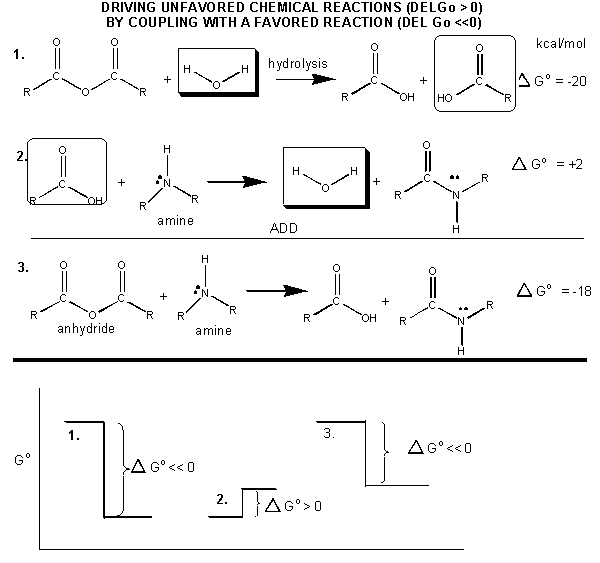# C1. ATP

Biological oxidation reactions serve two functions. Oxidation of organic molecules can produce new molecules with different properties. For example, increases in solubility is observed on hydroxylation of aromatic substrates by cytochrome P450. Likewise, amino acids can by oxidized to produce neurotransmitters. Most biological oxidation reactions occur, however, to produce energy to drive thermodynamically unfavored biological processes such as protein and nucleic acid synthesis, or motility. Chemical potential energy is not just released in biological oxidation reactions. Rather, it is transduced into a more useful form of chemical energy in the molecule ATP (adenosine triphosphate). This chapter will discuss the properties that make ATP so useful biologically, and how exergonic biological oxidation reactions are coupled to the synthesis of ATP.PROPERTIES OF ATP

ATP contains two phosphoanhydride bonds (connecting the 3 phosphates together) and one phosphoester bond (connecting a phosphate to the ribose ring). The pKa's for the reactions

$HATP^{3-} \rightarrow ATP^{4-} + H^+$

and

$HADP^{2-} \rightarrow ADP^{3-} + H^+$

are about 7.0, so the overall charges of ATP and ADP at physiological pH are -3.5 and -2.5, respectively. Each of the phosphorous atoms are highly electrophilic and can react with nucleophiles like the OH of water or an alcohol. As we discussed earlier, anhydrides are thermodynamically more reactive than esters which are more reactive than amides. The large negative $$ΔG^o = -7.5\, kcal/mol$$ for the hydrolysis (a nucleophilic substitution reaction) of one of the phosphoanhydride bonds can be attributed to a relative destabilization of the reactants (ATP and water) and relative stabilization of the products (ADP = Pi). Specifically

• The reactants can not be stabilized to the same extent as products by resonance due to competing resonance of the bridging anhydride O's.
• The charge density on the reactants is greater than that of the products
• Theoretical studies show that the products are more hydrated than the reactants.

The $$ΔG^o$$ for hydrolysis of ATP is dependent on the divalent ion concentration and pH, which affect the the stabilization and the magnitude of the charge states of the reactants and products.Jmol: Updated ATP Jmol14 (Java) | JSMol (HTML5) | ADP Jmol14 (Java) | JSMol (HTML5)Figure: STRUCTURE AND HYDROLYSIS OF ATP

Carboxylic acid anhydrides are even more unstable to hydrolysis than ATP (-20 kcal/mol), followed by mixed anhydrides (-12 kcal/mol), and phosphoric acid anhydrides (-7.5 kcal/mol). These molecules are often termed "high energy" molecules, which is somewhat of a misnomer. They are high energy only in relation to the energy of their cleavage products, such that the reaction proceeds with a large negative $$ΔG^o$$.Figure: HIGH ENERGY MOLECULES

How can ATP be used to drive thermodynamically unfavored reaction? First consider how the hydrolysis of a carboxylic acid anhydride, which has a $$ΔG^o$$ = -12.5 kcal/mol can drive the synthesis of a carboxylic acid amide, with a $$Δ G^o$$ of + 2-3 kcal/mol. The link below shows the net reaction, (anhydride + amine --> amide + carboxylic acid), which can be broken into two reactions: hydrolysis of the anhydride, and the synthesis of the amide.

Figure: MECHANISM: COUPLED SYNTHESIS OF A CARBOXYLIC AMIDENow consider the reaction of glucose + Pi to form glucose-6-P. In this reaction a phosphoester is formed, so the reaction would proceed with a positive ΔGo = 3.3. Now if ATP was used to transfer the terminal (gamma) phosphate to glucose to form Glc-6-P, the reaction proceeds with a ΔGo = -4 kcal/mol. This can be calculated since ΔG is a state function and is path independent. Adding the reactions and the ΔGo's for

$\ce{glucose + Pi -> glucose-6-P }$

and

$\ce{ATP + H2O -> ADP + Pi}$

gives the resultant reaction and $$ΔG^o$$

$\ce{glucose + ATP -> Glucose-6-P + ADP, }$

with $$ΔG^o = -4$$.

In most biological reactions using ATP, the terminal P of ATP is transferred to a substrate using an enzyme called a kinase. Hence, hexokinase transfers the gamma phosphate from ATP to a hexose sugar. Protein kinase is an enzyme which transfers the gamma phosphate to a protein substrate.

ATP is also used to drive peptide bond (amide) synthesis during protein synthesis. From an energetic point of view, anhydride cleavage can provide the energy for amide bond formation. Peptide bond synthesis is cells is accompanied by cleavage of both phosphoanhydride bonds in ATP in a complicated set of reactions that is catalyzed by ribosomes in the cells. (This topic is considered in depth in molecular biology courses). The figure below is a grossly simplified mechanism of how peptide bond formation can be coupled to ATP cleavage.

Figure: MECHANISM: ATP-DEPENDENT PEPTIDE BOND SYNTHESISPhosphorylation reactions using ATP are really nucleophilic substitution reactions which proceed through a pentavalent intermediate. The rest of the ATP molecule is then considered the leaving group, which could be theoretically ADP or AMP as well. If water is the nucleophile, the reaction is also a hydrolysis reaction. These reactions are also called phosphoryl transfer reactions.

One last note. ATP exists in cells as just one member of a pool of adenine nucleotides which consists of not only ATP, but also ADP and AMP (along with Pi). These constituents are readily interconvertible. We actually break down an amount of ATP each day equal to about our body weight. Likewise we make about the same amount from the turnover products. When energy is needed, carbohydrates and lipids are oxidized and ATP is produced, which can then be immediately used for motility, biosynthesis, etc. It is very important to realize that although ATP is converted to ADP in a thermodynamically spontaneous process, the process is kinetically slow without an enzyme. Hence ATP is stable in solution. However, its biological half-life is not long since it is used very quickly as described above. This recapitulates a theme we have seen before. Many reactions (like oxidation with dioxygen, denaturation of proteins in nonpolar solvent, and now ATP hydrolysis) are thermodynamically favored but kinetically slow. This kinetic slowness is a necessary but of course insufficient condition, for life.